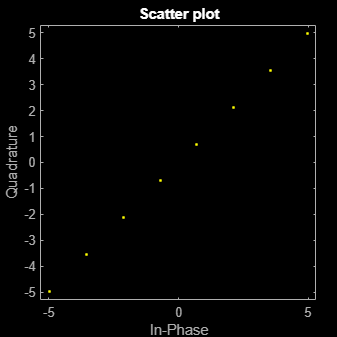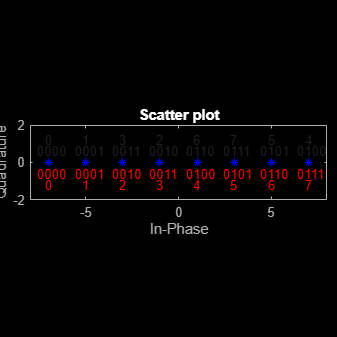# pammod

Pulse amplitude modulation

## Syntax

```y = pammod(x,M) y = pammod(x,M,ini_phase) y = pammod(x,M,ini_phase,symbol_order) ```

## Description

`y = pammod(x,M)` outputs the complex envelope `y` of the modulation of the message signal `x` using pulse amplitude modulation. `M` is the alphabet size. The message signal must consist of integers between 0 and `M-1`. The modulated signal has a minimum Euclidean distance of 2. If `x` is a matrix with multiple rows, the function processes the columns independently.

`y = pammod(x,M,ini_phase)` specifies the initial phase of the modulated signal in radians.

`y = pammod(x,M,ini_phase,symbol_order)` specifies how the function assigns binary words to corresponding integers. If `symbol_order` is set to `'bin'` (default), the function uses a natural binary-coded ordering. If `symbol_order` is set to `'gray'`, it uses a Gray constellation ordering.

## Examples

collapse all

Generate random data symbols and apply pulse amplitude modulation.

Set the modulation order.

`M = 8;`

Generate random integers and apply PAM modulation having an initial phase of $\pi$/4.

```data = randi([0 M-1],100,1); modData = pammod(data,M,pi/4);```

Display the PAM constellation diagram.

`scatterplot(modData)`Plot PAM symbol mapping for Gray and natural binary encoded data.

Set the modulation order, and then create a data sequence containing a complete set of constellation points.

```M = 8; data = [0:M-1];```

Modulate and demodulate Gray and natural binary encoded data.

```symgray = pammod(data,M,0,'gray'); mapgray = pamdemod(symgray,M,0,'gray'); symbin = pammod(data,M,0,'bin'); mapbin = pamdemod(symbin,M,0,'bin');```

Plot the constellation points using one of the symbol sets. For each constellation point, assign a label indicating the Gray and natural binary values for each symbol.

• For Gray binary symbol mapping, adjacent constellation points differ by a single binary bit and are not numerically sequential.

• For natural binary symbol mapping, adjacent constellation points follow the natural binary encoding and are sequential.

```scatterplot(symgray,1,0,'b*'); for k = 1:M text(real(symgray(k))-0.6,imag(symgray(k))+0.6,... dec2base(mapgray(k),2,4)); text(real(symgray(k))-0.2,imag(symgray(k))+1.2,... num2str(mapgray(k))); text(real(symbin(k))-0.6,imag(symbin(k))-0.6,... dec2base(mapbin(k),2,4),'Color',[1 0 0]); text(real(symbin(k))-0.2,imag(symbin(k))-1.2,... num2str(mapbin(k)),'Color',[1 0 0]); end axis([-M M -2 2])```